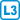## Choice variance

Let the vector $$\mathbf x=(x_1,…,x_n)\in \mathbb R^n$$ represents $$n$$ observations, i.e. choices. The variance is defined as $$\displaystyle \sigma^2=\sum_{i=1}^n (x_i-\bar x)^2$$, where $$\displaystyle \bar x=\frac 1n \sum_{i=1}^n x_i$$ is the average.

Show that $$\sigma^2$$ is a quadratic form on $$\mathbb R^n$$ and determine the matrix of this form.

• #### Resolution

We use the well known fact

$$\displaystyle \sigma^2=\sum_{i=1}^n (x_i-\bar x)^2= \sum_{i=1}^n (x_i^2-\bar x^2)$$,

hence

$$\displaystyle \sum_{i=1}^n (x_i^2-\bar x^2)= \sum_{i=1}^n x_i^2- n\bar x^2= \sum_{i=1}^n x_i^2- n\left(\frac 1n \sum_{i=1}^n x_i \right)^2= \sum_{i=1}^n x_i^2- \frac 1n \sum_{i=1}^n\sum_{j=1}^n x_ix_j=$$
$$\mathbf x^T\mathbf x -\frac1n \mathbf x^T\mathbf J_n\mathbf x= \mathbf x^T\left(\mathbf I_n-\frac 1n \mathbf J_n\right)\mathbf x$$,

where the matrix $$\mathbf J_n$$ contains only ones.

Note that the above relation can also be derived as:

$$\sum_{i=1}^n (x_i-\bar x)^2=\sum_{i=1}^n (x_i^2-2x_i\bar x+ \bar x^2)= \sum_{i=1}^n x_i^2 -2\bar x \sum_{i=1}^n x_i+ n\bar x^2=$$
$$\sum_{i=1}^n x_i^2 -n\bar x^2= \sum_{i=1}^n (x_i^2-\bar x^2)$$.

• #### Result

The form can be expressed as the product $$\sigma^2=\mathbf x^T\left(\mathbf I_n-\frac 1n \mathbf J_n\right)\mathbf x$$.

The matrix of the form is $$\begin{pmatrix} \frac{n-1}{n} & -\frac1n & … &-\frac1n \\ -\frac1n & \frac{n-1}{n} & \ddots & \vdots \\ \vdots &\ddots &\ddots &-\frac1n\\[3pt] -\frac1n & … & -\frac1n & \frac{n-1}{n} \\ \end{pmatrix}$$